Clutch Prep is now a part of Pearson
Ch.1 - Intro to General ChemistryWorksheetSee all chapters

# Significant Figures

See all sections
Sections
Classification of Matter
Physical & Chemical Changes
Chemical Properties
Physical Properties
Intensive vs. Extensive Properties
Temperature
Scientific Notation
SI Units
Metric Prefixes
Significant Figures
Significant Figures: Precision in Measurements
Significant Figures: In Calculations
Conversion Factors
Dimensional Analysis
Density
Density of Geometric Objects
Density of Non-Geometric ObjectsJules Bruno

Every measurement or calculation we do with instruments in chemistry has some level of uncertainty called experimental error. Significant figures are necessary to communicate a level of accuracy and precision while dealing with this uncertainty.

Rules for Number of Signficant Figures

Rule 1: If your number has a decimal point move from left to right. Start counting once you get to your first non-zero number and keep counting until you get to the end.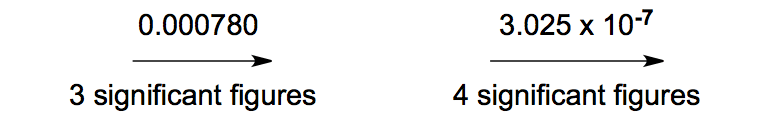Signficant Figures with Decimal Points

Trailing zeros are a sequence of zeros after the decimal point once you’ve passed all non-zero integers. They would be significant.

Rule 2: If your number has NO decimal point move from right to left. Start counting once you get to your first non-zero number and keep counting until you get to the end.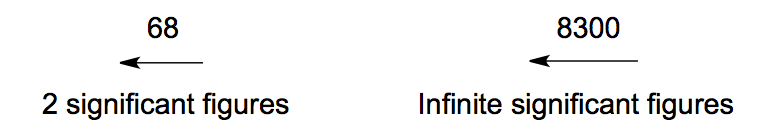Significant Figures (No Decimal Points)If a value has no decimal point then the final zero would mean it has an infinite number of significant figures.

Exact Numbers

Exact numbers contain an infinite number of significant figures because they represent the amount of a particular item and not a measurement.

For example, when we say that a decade is equal to 10 years, there are 150 students in your chemistry class, or NH3 contains 1 atom of nitrogen and 3 atoms of hydrogen.

When dealing with addition or subtraction the least number of decimal places will determine the final answer.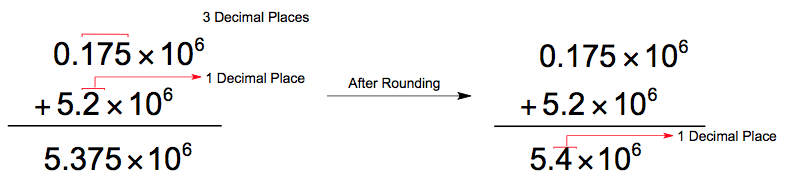Significant Figures (Addition)Adding the two values gives the initial value of 5.375 x 106, but the final answer should have only 1 decimal place and so the answer is rounded.

Multiplication & Division

When dealing with multiplication or division the least number of significant figures will determine the final answer.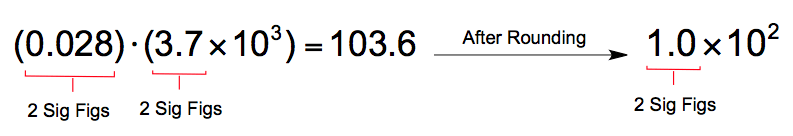Significant Figures (Multiplication)

Multiplying the two values gives an initial value of 103.6, but you must round your final answer to significant figures.

Furthermore keep in mind when dealing with mixed operations we must follow the same arithmetic guidelines as we always do.

Logarithm & Natural Logarithm

When  of a value, the number of significant figures it has determines the number of digits  after the decimal point for the answer.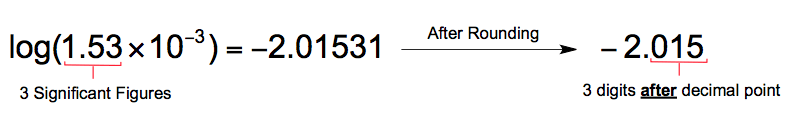Significant Figures (Logarithms)

Using your calculator, the log of 1.53 x 10-3 gives an initial value of - 2.01531. The value of 1.53 x 10-3 has 3 significant figures and so the final answer should have 3 digits after the decimal point.Jules Bruno

Jules felt a void in his life after his English degree from Duke, so he started tutoring in 2007 and got a B.S. in Chemistry from FIU. He’s exceptionally skilled at making concepts dead simple and helping students in covalent bonds of knowledge.